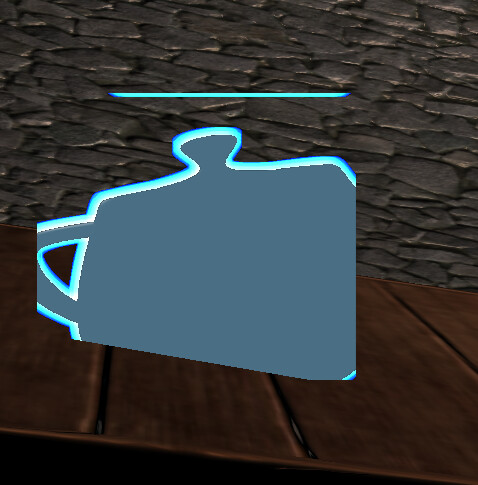# Rendering Model to Quad Properly

I’m new here so sorry if this is formatted poorly, I’ll edit it as needed

Basically, I asked my lecturer how to do this glowing effect, there’s a language barrier so understanding him didn’t really work out. I then asked some coursemates, who also don’t know how to do this step. Basically, we’ve got our models drawn to a render target, and then we’ve smoothed the object to add a glow effect.

This glowing object then gets rendered to a quad on the screen, but our issue is that as we rotate around in the world, the object doesn’t rotate/move as it should, sometimes it clips outside the quad, if I zoom in too far - it will also get too large and fill up past the boundaries of the quad.

I have a feeling it’s either to do with which space I’m rendering the initial object in, or perhaps I’m missing something in my calculation for billboarding, although I thought I made it correct based on our lecture slides. Here is the vertex shader that handles billboarding

``````varying vec2 TexCoord;
uniform mat4 invView;
uniform mat4 scale;
uniform vec3 translate;

void main(void)
{
vec3 pos = gl_Vertex.xyz;

mat4 iView = invView * scale;
vec3 Vx = iView.xyz;
vec3 Vy = iView.xyz;
vec3 Vz = iView.xyz;
pos = (pos.x * Vx + pos.y * Vy + pos.z * Vz).xyz;
gl_Position = gl_ModelViewProjectionMatrix * vec4(pos,1.0);
TexCoord = gl_MultiTexCoord0.xy;
}
``````Thanks for any help guys, I’m new here again so sorry if I haven’t included everything I should

The main thing to consider with billboarding is that the composition of the transformation used for rendering the object onto the quad and the transformation used when rendering the quad onto the screen should be (approximately) equal to the transformation you would use for rendering the object directly onto the screen. Note that this includes all of the transformations, including the texture and viewport transformations. Don’t forget that NDC is [-1,1] while texture coordinates are [0,1]. Finally, the quad must be large enough to cover the object, otherwise it will be clipped.

Okay, I thought it could’ve been the composition of all the transformations, I’ll try figuring out how to reduce them to get it closer to the transform the model would be under if it weren’t being drawn onto a quad.

thanksHi again, sorry to be a pain

Could I get some more guidance on how to ensure the composite transformations acting on the model and quad are roughly the same as if it was just model?

Is there a way I can, for example, isolate the rotation and apply that to the object from the view, disregarding the translation? So the object stays at (0,0,0) and then the billboard is responsible for translation?

I’m a bit unsure how to do this

Many thanks

If the quad is constructed so that it appears as a rectangle when rendered (and it usually should), the difference between the transformation used when rendering the object onto the quad and rendering the quad onto the window is essentially a viewport transformation.

If the quad forms the rectangle [x0,x1]×[y0,y1] in NDC, then you need to prepend a transformation

``````[sx  0  0 tx]
[ 0 sy  0 ty]
[ 0  0  1  0]
[ 0  0  0  1]
``````

where
sx = 2/(x1-x0)
sy = 2/(y1-y0)
tx = -(x0+x1)/(x1-x0)
ty = -(y0+y1)/(y1-y0)

More generally, if the NDC (x,y) coordinates of 3 of the vertices are (x0,y0), (x1,y1), (x2,y2) (for the lower-left, lower-right and upper-left respectively), then set

``````V = [x0 x1 x2]
[y0 y1 y2]
[ 1  1  1]

N = [-1  1 -1]
[-1 -1  1]
[ 1  1  1]
``````

and the required transformation is T·V=N => T=N·V-1

If the quad isn’t face-on to the viewpoint, then to get a reasonable result you’d need to use homogeneous texture coordinates (s,t,1,q) when rendering the quad.

1 Like

Thank you for that information, it’s super helpful

I’ve still barely scratched the surface of shaders and OpenGL itself, so I’m still getting familiarised with all the different spaces and matrix multiplications